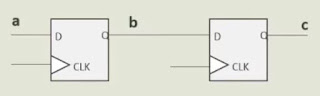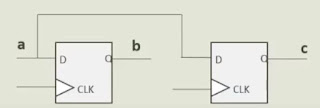### Modelling Sequential and Combinational Circuits: Part-2

BLOCKING AND NON-BLOCKING ASSIGNMENTS: The statements in 'always' block use either blocking or non-blocking assignment.

• Blocking assignments: =
• In Simulation, all statements within an 'always' block are executed sequentially every time the block is evaluated.
• The always block is evaluated either in every clock (sequential logic) OR when there's a change in sensitivity list (combinational logic).
• Right-side of the first expression is evaluated, assigned to the left side, goes to the left side and does the same thing.
• In Synthesis, the tool will generate the circuit inside 'always' block in an equivalent manner to evaluate all statements sequentially.
• Non-Blocking assignments: <=
• In Simulation, all statements within an 'always' block are executed in parallel every time the block is evaluated. Which precisely means, Right side expressions are evaluated using the values from the previous time slot and they are assigned to the left side simultaneously.
• In Synthesis, the tool will generate the circuit inside 'always' block in an equivalent manner to evaluate all the statements in parallel.
• Blocking and Non-Blocking assignments - Simulation:
• Blocking assignments =
• Right side of first expression is evaluated, assigned to its Left side and goes to its next statement and so on.
• Order of expression matters. (See in the below example)
e.g.

always @ (posedge clk) begin
c = b;
b = a;
end

/*
assume at some clock, values of a and b were a=2 b=8.
Then in the next clock
c=8 : as the value of c is immediately assigned to that of b
b=2 : as the value of b is immediately assigned to that of a
*/
always @ (posedge clk) begin
b = a;
c = b;
end

/*
assume at some clock, values of a and b were a=2 b=8.
Then in the next clock
b=8 : as the value of b is immediately assigned to that of a
c=2 : as the value of c is immediately assigned to that of b
*/

• Non-Blocking assignments <=
• All Right side expressions are evaluated simultaneously using the previous timeslot, and then they are assigned to the Left side while exiting from the timeslot simultaneously.
• Order of the expression doesn't matter.
e.g.

always @ (posedge clk) begin
c <= b;
b <= a;
end

always @ (posedge clk) begin
b <= a;
c <= b;
end

/*
assume at some clock, values of a and b were a=2 b=8.
Then in the next clock
c=8 : as the value of c is assigned to that of b in the "previous" clock
b=2 : as the value of b is assigned to that of a in the "previous" clock
*/
• Blocking and Non-Blocking assignments - Synthesis:
• Blocking assignments =
• In simulation, all statements within an always block are executed sequentially every time the block is evaluated.
• In synthesis, the tool will generate the circuit inside always block in an equivalent manner to evaluate all the statements sequentially.
always @ (posedge clk) begin
c = b;
b = a;
endFig: flipflop representation of the above code

always @ (posedge clk) begin
b = a;
c = b;
endFig: flipflop representation of the above code
• Non-Blocking assignments <=
• In simulation, all statements within an always block are executed in parallel every time the block is evaluated.
• In synthesis, the tool will generate the circuit inside always block in an equivalent manner to evaluate all the statements in parallel.
always @ (posedge clk) begin
c <= b;
b <= a;
endFig: flipflop representation of the above code

always @ (posedge clk) begin
b <= a; // the value of b equals prev value of a
c <= b; // the value of c equals prev value of b
endFig: flipflop representation of the above code

Implies, same circuit is generated even after changing the order.

• Guidelines for using Blocking and Non-Blocking assignments:
• When modelling a sequential circuit, use non-blocking assignments. <=
• When modelling latches, use non-blocking assignments. <=
• When modelling combinational logic with an always block, use blocking assignments. =
• When modelling both sequential and combinational logic within the same always block, use non-blocking assignments. <=
• Do not mix blocking and non-blocking assignments in the same always block.
• Do not make assignments to the same variable for more than one time in the same always block.
*Following the above guidelines will accurately model synthesizable hardware while eliminating 90-100% of the most common Verilog simulation race conditions.